Question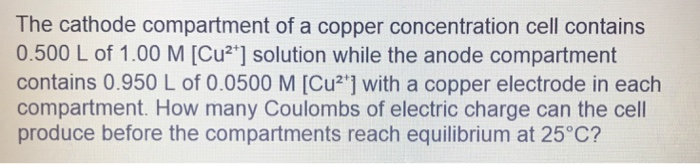We need at least 10 more requests to produce the answer.

0 / 10 have requested this problem solution

The more requests, the faster the answer.

All students who have requested the answer will be notified once they are available.

#### Earn Coins

Coins can be redeemed for fabulous gifts.

Similar Homework Help Questions
• ### The cathode compartment of a copper concentration cell contains 0.500 L of 1.00 M [Cu2+] solution...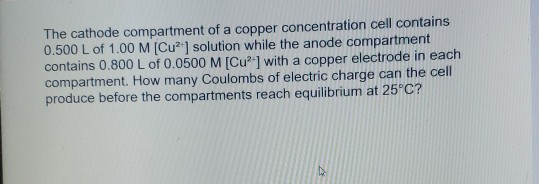The cathode compartment of a copper concentration cell contains 0.500 L of 1.00 M [Cu2+] solution while the anode compartment contains 0.800 L of 0.0500 M [Cu] with a copper electrode in each compartment. How many Coulombs of electric charge can the cell produce before the compartments reach equilibrium at 25°C?

• ### The cathode compartment of a copper concentration cell contains 0.500 L of 1.00 M (Cu*] solution...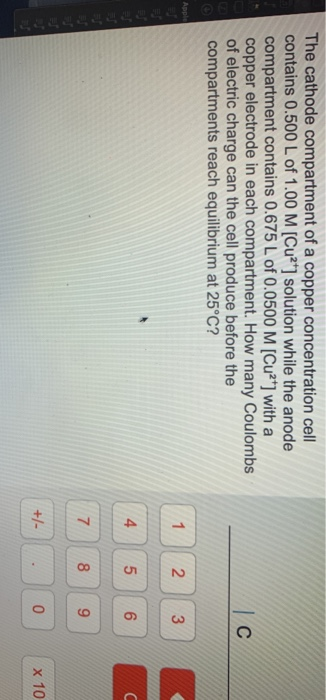The cathode compartment of a copper concentration cell contains 0.500 L of 1.00 M (Cu*] solution while the anode compartment contains 0.675 L of 0.0500 M [Cu2+] with a copper electrode in each compartment. How many Coulombs of electric charge can the cell produce before the compartments reach equilibrium at 25°C? с Apple 1 2 3 4 5 U 6 7 8 9 +1 0 x 10

• ### 3 ques plz help thank you The cathode compartment of a copper concentration cell contains 0.500...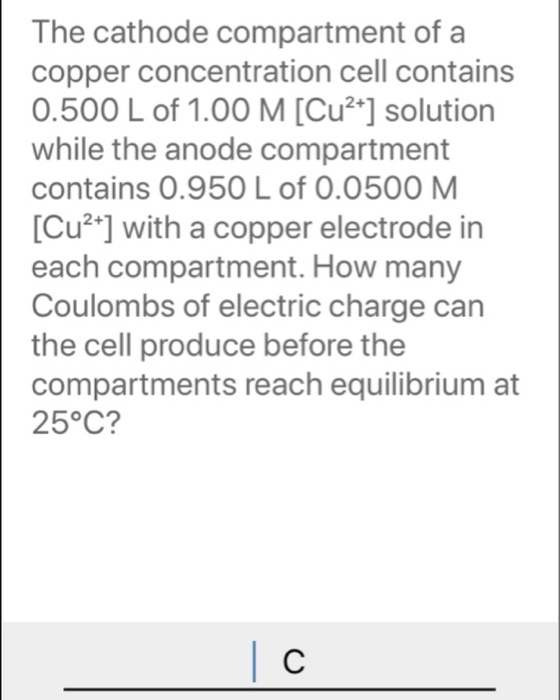3 ques plz help thank you The cathode compartment of a copper concentration cell contains 0.500 L of 1.00 M [Cu2+] solution while the anode compartment contains 0.950 L of 0.0500 M. [Cu2+] with a copper electrode in each compartment. How many Coulombs of electric charge can the cell produce before the compartments reach equilibrium at 25°C? | C Calculate the atomic mass of the magnesium-27 nucleus in amu (to three decimal places) assuming that the mass of a nucleon...

• ### need help plz 3 ques What is the correct systematic name for the compound shown here?...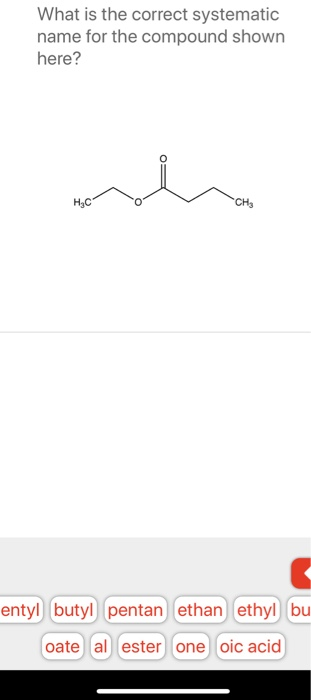need help plz 3 ques What is the correct systematic name for the compound shown here? HEC CHE entyl butyl pentan ethan ethyl bu oate al ester one oic acid The cathode compartment of a copper concentration cell contains 0.500 L of 1.00 M [Cu2+] solution while the anode compartment contains 0.950 L of 0.0500 M. [Cu2+] with a copper electrode in each compartment. How many Coulombs of electric charge can the cell produce before the compartments reach equilibrium at...

• ### *A copper, Cu(s), electrode is immersed in a solution that is 1.00 M in ammonia, NH3, and 1.00 M in tetraamminecopper(II), [Cu(NH3)4]2+. If a standard hydrogen electrode is used as the cathode, the ce...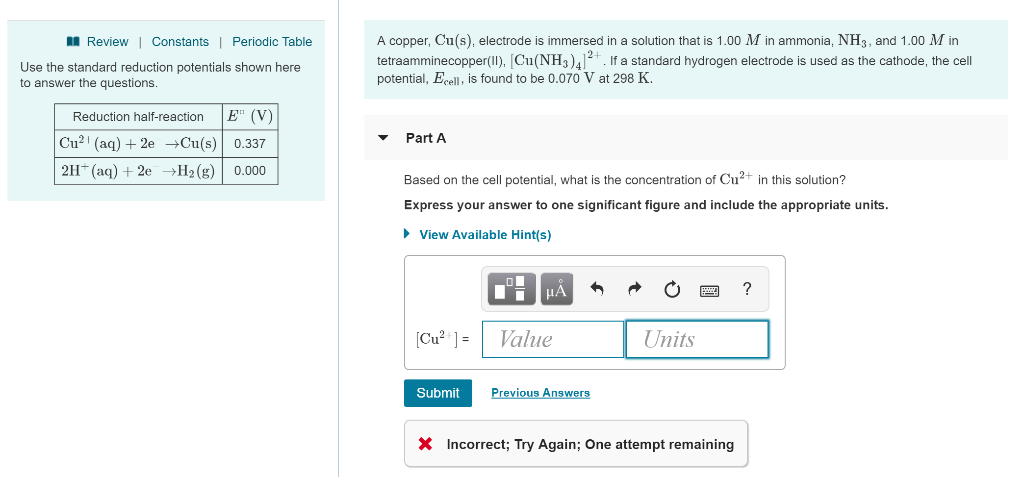*A copper, Cu(s), electrode is immersed in a solution that is 1.00 M in ammonia, NH3, and 1.00 M in tetraamminecopper(II), [Cu(NH3)4]2+. If a standard hydrogen electrode is used as the cathode, the cell potential, Ecell, is found to be 0.070 V at 298 K. A copper, Cu(s), electrode is immersed in a solution that is 1.00 M in ammonia, NH3, and 1.00 M in tetraamminecopper(II), [NH. If a standard hydrogen electrode is used as the cathode, the cell potential,...

• ### 1. Calculate the pH of the cathode compartment solution if the cell emf at 298 K...

1. Calculate the pH of the cathode compartment solution if the cell emf at 298 K is measured to be 0.610 V when [Zn2+]= 0.20 M and PH2= 0.92 atm . Express your answer using two decimal places. pH= Switch 8.76 Voltmeter 7n anode NO, Na +H (8) Cathode compartment (standard hydrogen electrode) Anode compartment NO, Zn2+ NO NO, Zn(s) —> Zn2+ (aq)+ 2e 2H+ (aq) + 2e →H(8)

• ### salt bridge ME Cr(s) electrode Cu(s) electrode 1.0 M Cr3+ (aq) 1.0 M Cu2+ (aq) A...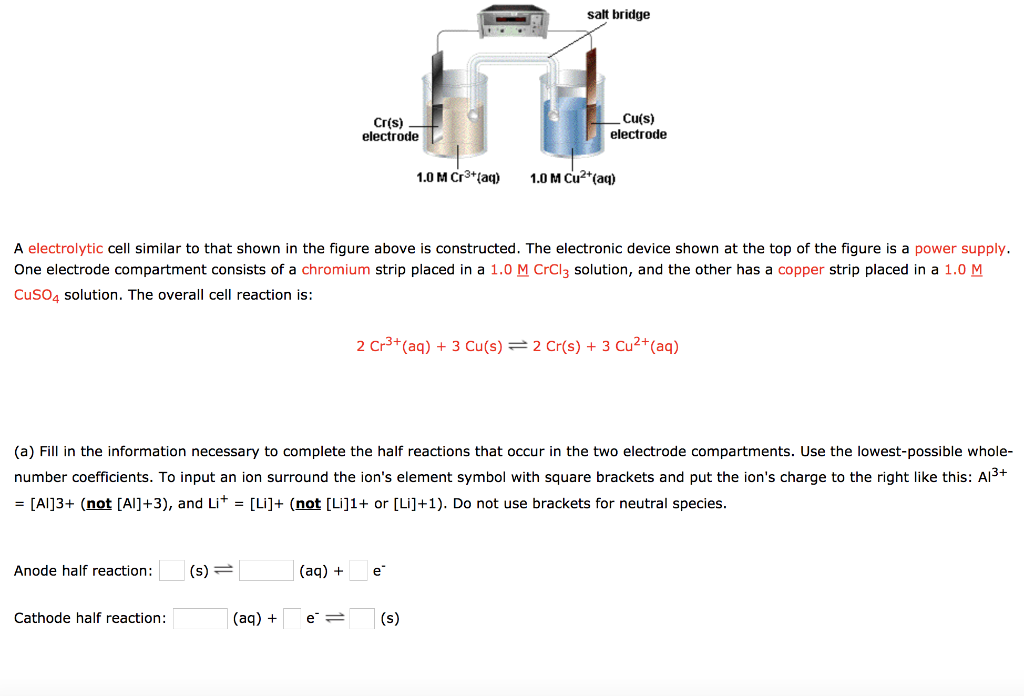salt bridge ME Cr(s) electrode Cu(s) electrode 1.0 M Cr3+ (aq) 1.0 M Cu2+ (aq) A electrolytic cell similar to that shown in the figure above is constructed. The electronic device shown at the top of the figure is a power supply. One electrode compartment consists of a chromium strip placed in a 1.0 M CrCl3 solution, and the other has a copper strip placed in a 1.0 M CuSO4 solution. The overall cell reaction is: 2 Cr3+ (aq) +...

• ### 14.__/15 pts) In a concentration cell, a voltage can be created when one compartment has a...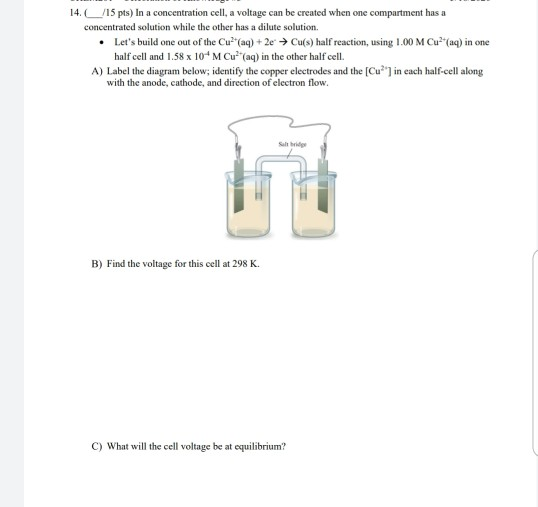14.__/15 pts) In a concentration cell, a voltage can be created when one compartment has a concentrated solution while the other has a dilute solution. • Let's build one out of the Cu?"(aq) + 2e → Cu(s) half reaction, using 1.00 M Cu?"(aq) in one half cell and 1.58 x 10 M Cu?"(aq) in the other half cell. A) Label the diagram below; identify the copper electrodes and the [Cu?") in each half-cell along with the anode, cathode, and direction...

• ### Part A Calculate the pH of the cathode compartment solution if the cell emf at 298...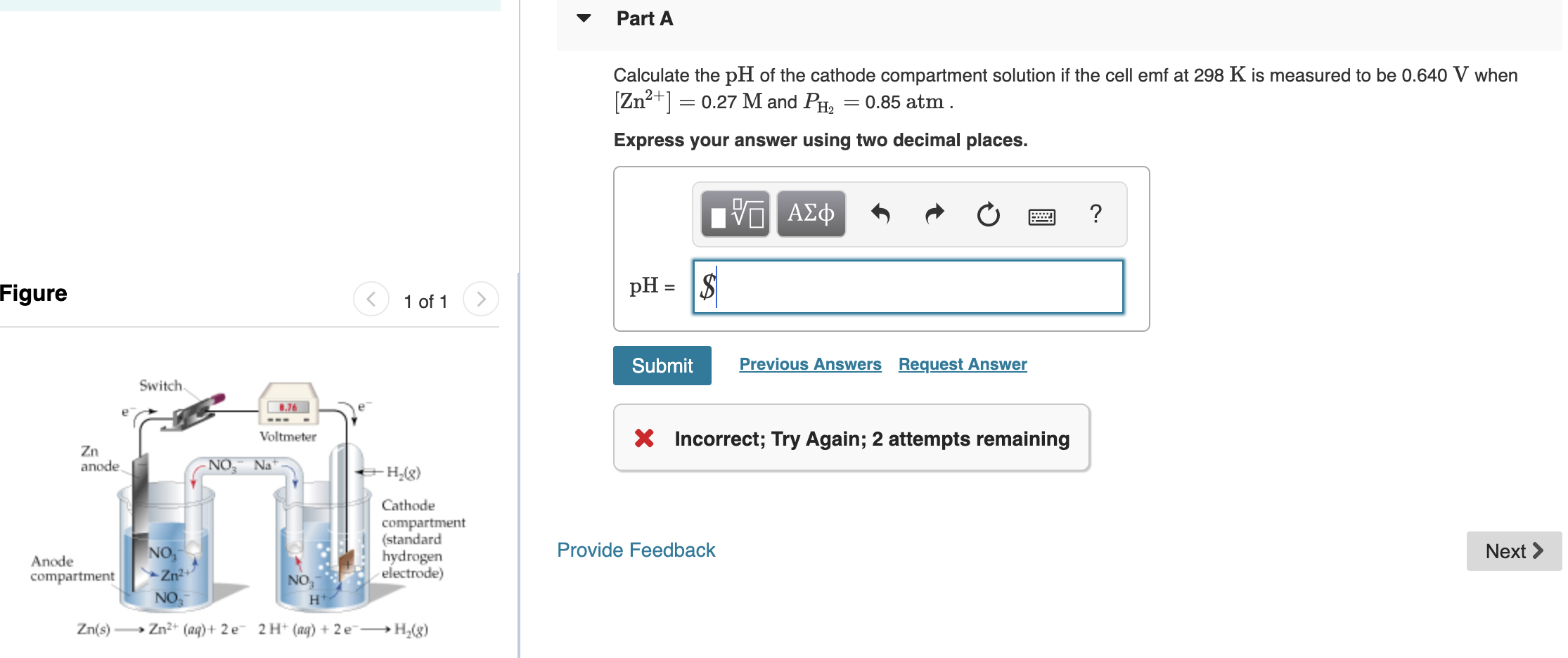Part A Calculate the pH of the cathode compartment solution if the cell emf at 298 K is measured to be 0.640 V when [Zn240.27 M and PH = 0.85 atm. Express your answer using two decimal places. V ΑΣφ pH =\$ Figure 1 of 1 Previous Answers Request Answer Submit Switch 8.76 X Incorrect; Try Again; 2 attempts remaining Voltmeter Zn anode NO Na - H2(g) Cathode compartment (standard hydrogen electrode) NO3 Zn2 Provide Feedback Next Anode compartment NO3...

• ### A galvanic cell consists of a manganese anode immersed in a MnSO4 solution and a copper cathode i...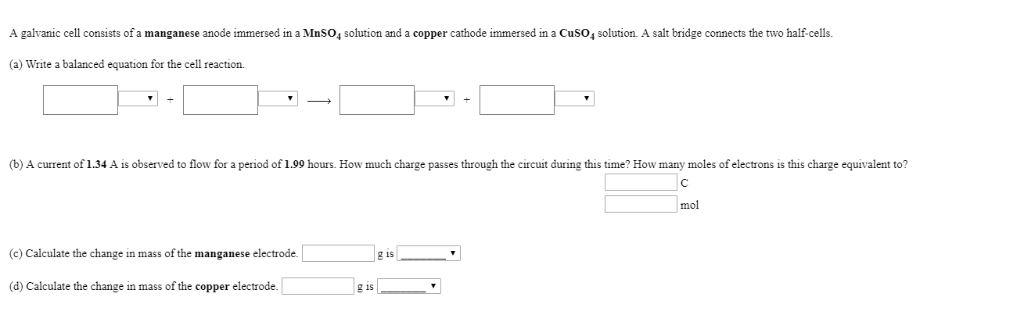A galvanic cell consists of a manganese anode immersed in a MnSO4 solution and a copper cathode immersed in a CuSo4 solution. A salt bridge connects the two half-cells (a) Write a balanced equation for the cell reaction. (b) A current of 1.34 A is observed to flow for a period of 1.99 hours. How much charge passes through the circuit during this time? How many moles of electrons is this charge equivalent to? mol (c) Calculate the change in...